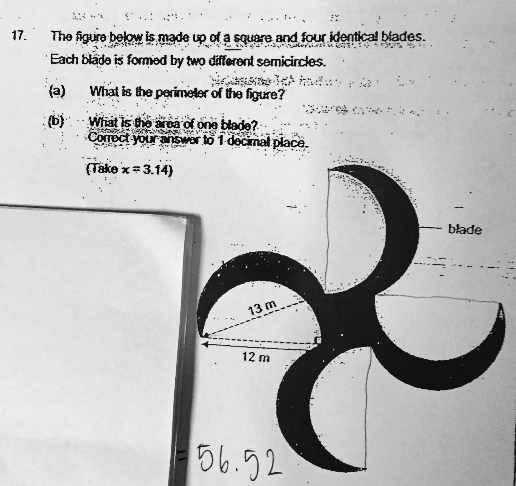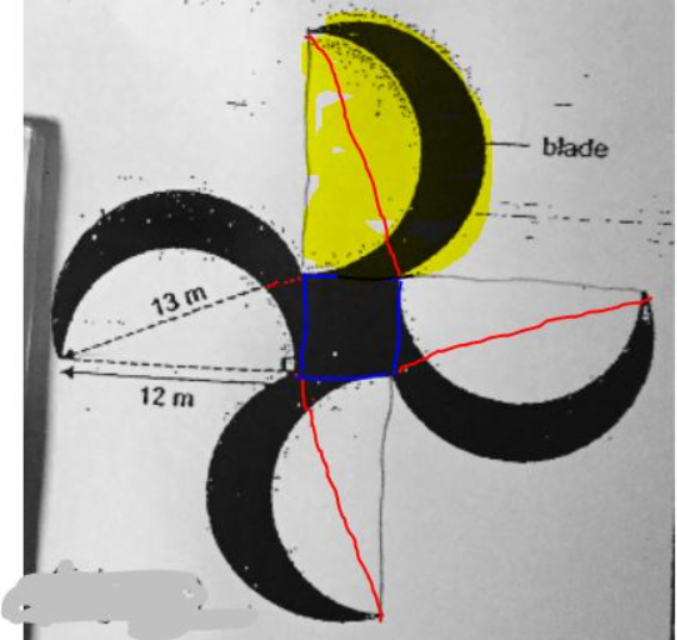# QuestionAnyone help me with attached question

Thanks

The figure below is made up of a square and four identical blades. Each blade is formed by two different semicircles. (a) What if the perimeter of the figure? (b)  (Given that the height of the triangle is 5 cm,) What is the area of one blade? Correct your answer to 1 decimal place. (Take pi = 3.14)(a)
perimeter of the figure ——- 4 x (1/2) x 3.14 x (12 + 13) = 157

(b)
area of one blade ——- (1/2) x 3.14 x (6.5 x 6.5 – 6 x 6) + (1/2) x 12 x 5 = 39.8125 = 39.8 (1 d.p.)

Ans : (a) 157 cm; (b)  39.8 sq cm (1 d.p.).

0 Replies 1 Like ✔Accepted AnswerPerimeter of figure = circumference of (4 big semi-circles + 4 small semi-circles)

=2 x 3.14 x 13 + 2 x 3.14 x 12

=157 cm#

Area of one blade (see yellow highlight)

= 1 right-angle triangle + 13-cm diameter semicircle – 12-cm diameter semicircle

= 1/2 x 12 x 5*                + 1/2 x 3.14 x 6.5                 – 1/2 x 3.14 x 62

= 39.8 sq cm*

Note*: 5cm is not shown on the diagram and I worked it out using pythagoras theorem (not for P6). This 5cm is most likely provided in diagram at first but now missing after all the “touch up” or printing of the exam papers. My guess only. Please counter check model answer.